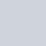# What is Stock Average Calculator?Published

on

A stock average calculator is a tool used to calculate the average price of a set of stocks. It’s a simple tool that takes into account the total value of your stocks and the total number of shares you own.

By inputting the current market value and the number of shares you own for each of your stocks into a stock average calculator, you can quickly and easily calculate the average price per share for your entire stock portfolio.

This can be helpful for investors who want to track the performance of their stocks over time or make decisions about buying or selling stocks based on their current average price.

Some stock average calculators may also include other features, such as the ability to calculate the total value of your stock portfolio or the rate of return on your investments.

## How Does Stock Average Calculator Works ?

A stock average calculator works by taking the total value of your stocks and the total number of shares you own and calculating the average price per share.

Here are the steps a stock average calculator typically follows:

Inputting Data, you input the current market value and the number of shares you own for each stock into the calculator.

Total Value Calculation, calculator multiplies the current market value by the number of shares you own for each stock to calculate the total value of each stock.

Total Shares Calculation, The calculator adds up the total number of shares you own for all your stocks.

Average Price Calculation, calculator divides the total value of your stock portfolio by the total number of shares you own to calculate the average price per share of your stock portfolio.

## Conclusion

In conclusion, a stock average calculator is a valuable tool for stock market investors. By providing an accurate picture of the average cost of stock investments, it allows investors to make informed investment decisions, track the performance of their stock portfolio, and plan for taxes more efficiently. Whether you are a seasoned investor or just starting out, a stock average calculator is an indispensable tool for your stock market journey.